There are 366 different Starters of The Day, many to choose from. You will find below some starters on the topic of Vocabulary. A lesson starter does not have to be on the same topic as the main part of the lesson or the topic of the previous lesson. It is often very useful to revise or explore other concepts by using a starter based on a totally different area of Mathematics.

Main Page

Vocabulary Starters:When written as a word or words what is the smallest positive whole number containing the letter 'a'?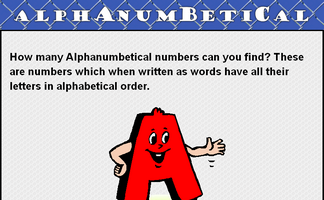A challenge to find the number which when written as a word has all the letters in alphabetical order.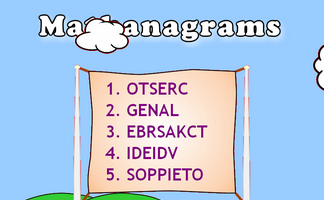Can you un-jumble these mathematical words?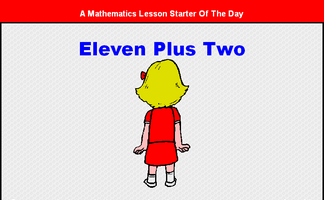Rearrange the letters in the expression 'Eleven Plus Two' to make another expression with the same value.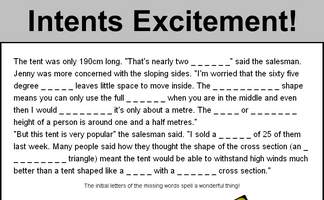Work out the mathematical words missing from the story about buying a tent.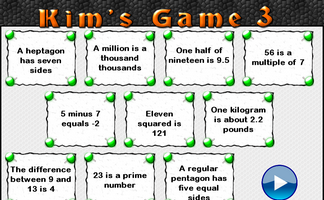This is the Maths version of the traditional memory game. Memorise 10 mathematical facts then recall them as they disappear from view.Find the nine mathematical words hidden in the love story. The last letters of the hidden words form an anagram of the name of a famous mathematician.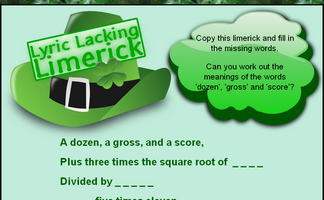Find the words missing from the mathematical limerick.Write down as many mathematical words as possible that begin with the letter M.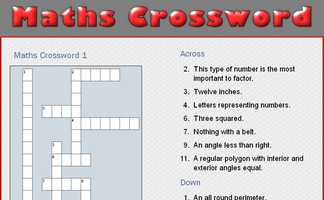A small crossword puzzle containing mathematical words.Find mathematical words which can be made by using the letters in the name of your school?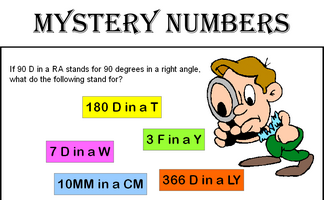Can you recognise the mystery numbers from the clues?Write down the names of all the mathematical shapes you know.Write down as many mathematical words as possible that begin with a particular letter.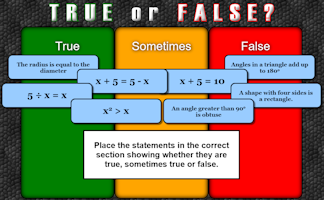An activity designed to address common misconceptions.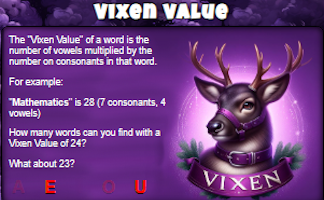The Verruca Value of a word is the number of vowels multiplied by the number of consonants. How many words can you find with Verruca Value of 24?Vowels have been taken out of mathematical words. Can you recognise them?Create your own mathematical word search for the whole class to enjoy.Turn mathematical words into designs which will help you remember the meanings of the words.

Search

The activity you are looking for may have been classified in a different way from the way you were expecting. You can search the whole of Transum Maths by using the box below.

Have today's Starter of the Day as your default homepage. Copy the URL below then select
Tools > Internet Options (Internet Explorer) then paste the URL into the homepage field.

Set as your homepage (if you are using Internet Explorer)

Do you have any comments? It is always useful to receive feedback and helps make this free resource even more useful for those learning Mathematics anywhere in the world. Click here to enter your comments.For All: